## 5. Hyperbolic Geometry

Hyperbolic geometry is the geometry you get by assuming all the postulates of Euclid, except the fifth one, which is replaced by its negation.

In hyperbolic geometry there exist a line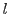and a point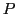not onsuch that at least two distinct lines parallel topass through.

It is more difficult to imagine, but have in mind the following image (and imagine that the lines never meet):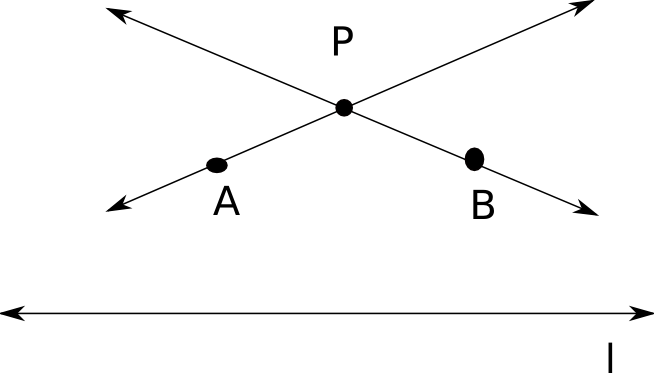The first property that we get from this axiom is the following lemma (we omit the proof, which is a bit technical):

Lemma 1   Rectangles don't exist in hyperbolic geometry.

Using this lemma, we can prove the following Universal Hyperbolic Theorem:

Theorem 1   In hyperbolic geometry, for every lineand every pointnot onthere pass throughat least two distinct parallels through. Moreover there are infinitely many parallels tothrough.

PROOF.

Drop the perpendicular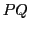toand erect a line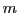throughperpendicular to, like in the figure below.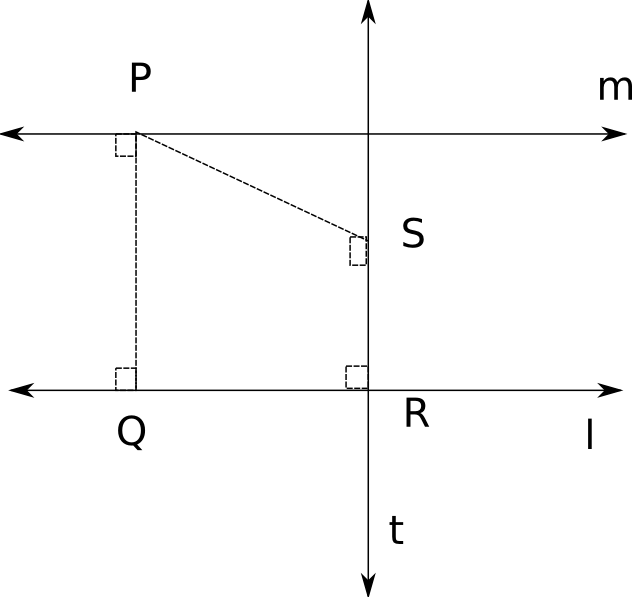Let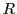be another point on, erect perpendiculartothroughand drop perpendicular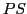to. Nowis parallel to, since both are perpendicular to.

Assume thatandare the same line (so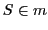). This would mean that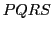is a rectangle, which contradicts the lemma above. Hence there are two distinct parallels tothrough. By varying, we get infinitely many parallels.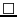Other important consequences of the Lemma above are the following theorems:

Theorem 2   In hyperbolic geometry, all triangles have angle sum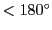. (From this it follows immediately that all convex quadrilaterals have angle sum less than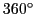).

Theorem 3   In hyperbolic geometry if two triangles are similar, they are congruent.

Note: This is totally different than in the Euclidean case. It tells us that it is impossible to magnify or shrink a triangle without distortion.

PROOF.

Assume the contrary: there are triangles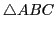and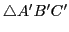that are similar (they have the same angles), but are not congruent. The no corresponding sides are congruent (otherwise, they would be congruent, using the principle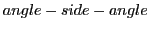). We may assume, without loss of generality, that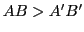and.Then, by definition of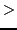there exists a point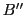on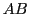and a point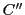on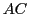such that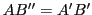and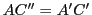. Then, since the angles are the same, by,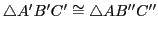. Hence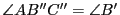and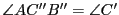.

But we also have that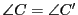and, so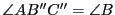and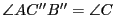. This implies that the linesand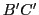are parallel, hence the quadrilateral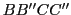is convex, and the sum of its angles is exactly, which contradicts the theorem above.

Hence similar triangles are congruent.#### Defect and area of a triangle

There is also the concept of the defect of a triangle, which is defined to be the quantity that is missing for the sum of the angles to add up to 180 degrees. And in fact the area of a triangle in hyperbolic geometry is proportional to its defect D. So we have that A=kD, where k is a positive number.
Two triangles have the same area if and only if they have the same angle-sum.

Now that you have experienced a flavour of proofs in hyperbolic geometry, Try some exercises!

#### Exercises

The following are exercises in hyperbolic geometry. You are to assume the hyperbolic axiom and the theorems above.

1. Prove that if A'B'BA is a Saccheri quadrilateral (the angles A' and B' are right angles and AA'=BB'), then the summit AB is greater than the base A'B'. (Hint: Join the midpoints M and M')
2. Suppose that lines l and l' have a common perpendicular MM'. Let A and B be points on l such that M is not the midpoint of AB. Show that A and B are not equidistant froml'.
3. Suppose that the parallel lines l and l' have a common perpendicular MM'. Prove that MM' is the shortest segment between any point of l and any point of l'.
4. Suppose that the parallel lines l and l' have a common perpendicular MM'. Let A and B be points on l such that A is between M and B. Drop perpendiculars AA' and BB' to l'. Prove that AA'<BB'.
5. Is every Saccheri quadrilateral a convex quadrilateral? Why or why not?

### Back to Escher

M. C. Escher created four patterns using hyperbolic geometry: Circle Limit I, Circle Limit III, Circle Limit III and Circle Limit IV. What Escher used for his drawings is the Poincaré model for hyperbolic geometry.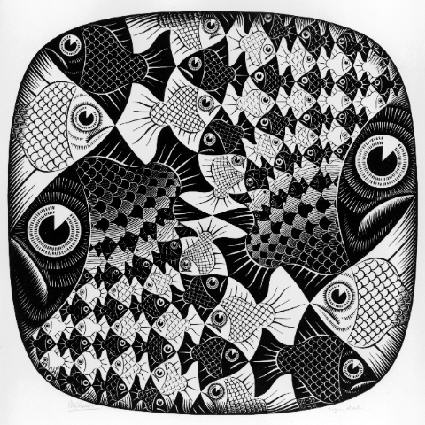"Circle Limit I" "Circle Limit II"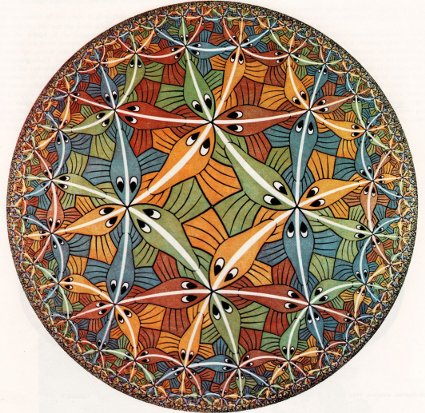"Circle Limit III" "Circle Limit IV"

What does it mean a model? By a model, we mean a choice of an underlying space, together with a choice of how to represent basic geometric objects, such as points and lines, in this underlying space. The need to have models for the hyperbolic plane (or better said, the hyperbolic geometry of the plane) is that it is very difficult to work with an Euclidean representation, but do non-Euclidean geometry. As you saw above, it is difficult to picture the notions that we work with, even if the proofs follow logically from our assumptions.

There are two more popular models for the hyperbolic plane: the upper half-plane model and the Poincaré plane model. We will analyse both of them in the following sections.

 INDEX NEXT